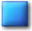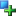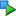##Image_Graph_Axis_Values

Description

Class for handling textual and numerical values on the axes

This class provied methods and attributes for customization of an axis. You can use it to set the options like the font, it's size or the color in which values (both numerical and textual, depending on the axis) will be drawn. For a numerical axis this class also offers you functionality to set the format in which numbers will be drawn. You can use this to set the number of decimal digits, prepend or append text etc. For a textual axis (actually: for the x-axis) this class allows you to set the text-labels which will be drawn.

Located in /Graph/Elements.php (line 922)

```Image_Graph_Base
|
--Image_Graph_Title
|
--Image_Graph_Axis_Values```
Method SummaryImage_Graph_Axis_Values Image_Graph_Axis_Values ()void setNumberformat (string \$format)void setText (array \$texts)
MethodsConstructor Image_Graph_Axis_Values (line 937)

Constructor

• access: public
Image_Graph_Axis_Values Image_Graph_Axis_Values ()setNumberformat (line 958)

Set numberformat for numerical values on the axis

Use this to set the format in which numbers on the axis will be drawn. E.g. you can use it to set a certain number of decimal values or prepend/append text to the numbers. Numbers will be drawn at all "major ticks" on the axis. Note that the "numberformat" is only used if the type of the axis is numerical (linear or at some later step even logarithmic), not if it's a textual axis (x-axis).

• access: public
void setNumberformat (string \$format)
• string \$format: sprintf-formatstringsetText (line 974)

Set text-labels on axis

These text-labels will be used (shown) when the axis-type is IMAGE_GRAPH_AXISTYPE_TEXT. The array contains one string per data-column. Multiple lines are possible by using "\n" as separator.

• access: public
void setText (array \$texts)
• array \$texts: (array of string) Text-labels

Redefinition of:
Image_Graph_Base::setText()
Set text

#### Inherited Methods

Inherited From Image_Graph_TitleImage_Graph_Title::Image_Graph_Title()Image_Graph_Title::setColor()

Inherited From Image_Graph_BaseImage_Graph_Base::setColor()Image_Graph_Base::setFontOptions()Image_Graph_Base::setSpacer()Image_Graph_Base::setText()

Documentation generated on Sat, 21 Feb 2004 23:28:03 +0100 by phpDocumentor 1.3.0RC2